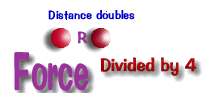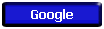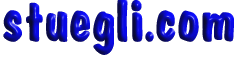# -

 Notes Outline What we are going to learn The Universal Law of Gravitation How two objects with mass attract each other Students should be able to recognize application and examples of the inverse square law What we know centripetal acceleration The Discovery of Gravity The Falling Apple The Falling Moon The Falling Earth The Universal Gravitation Constant, 'G' Things that follow this law will grown smaller by the square of the distance between them double distance and it it will be 4 times smallertriple distance and it 9 times smaller or 1/9 of the previous value     Universal Gravitation Perturbation LINKS TO MORE STUFF HISTORY Galileo's Actual Manuscript Notes on Motion (do you know Latin? if you know Spanish you may understand some of this!) Tutorials and more explanations The physics hypertextbook - universal gravitation http://hypertextbook.com/physics/mechanics/gravitation/ HyperPhysics - Gravity Gravity Sims Interactive 3D Solar System Sim (download file , right click and select 'install') (I haven't tried these yet, let me know if any of them are good!) http://www.handyarchive.com/free/gravity/Copyright © 2005 -  S. B. EglI